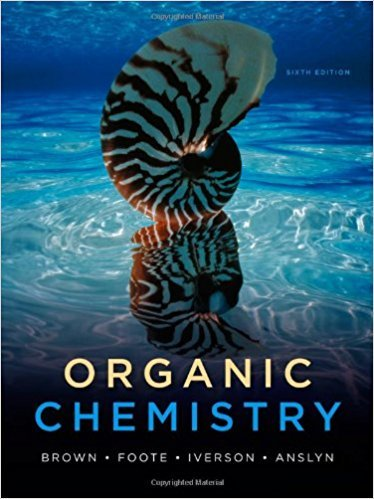×
×

# Solutions for Chapter 10: Organic Chemistry 6th Edition## Full solutions for Organic Chemistry | 6th Edition

ISBN: 9780840054982Solutions for Chapter 10

Solutions for Chapter 10
4 5 0 299 Reviews
25
5
##### ISBN: 9780840054982

Since 56 problems in chapter 10 have been answered, more than 38295 students have viewed full step-by-step solutions from this chapter. This textbook survival guide was created for the textbook: Organic Chemistry, edition: 6. This expansive textbook survival guide covers the following chapters and their solutions. Chapter 10 includes 56 full step-by-step solutions. Organic Chemistry was written by and is associated to the ISBN: 9780840054982.

Key Chemistry Terms and definitions covered in this textbook
• 1,2-elimination

An elimination reaction in which a proton from the beta (b) position is removed together with the leaving group, forming a double bond.

• amphoteric oxides and hydroxides

Oxides and hydroxides that are only slightly soluble in water but that dissolve in either acidic or basic solutions. (Section 17.5)

• Carbene

A neutral molecule that contains a carbon atom surrounded by only six valence electrons (R2C:).

• chemical reactions

Processes in which one or more substances are converted into other substances; also called chemical changes. (Section 1.3)

• Covalent bond

A chemical bond formed between two atoms by sharing one or more pairs of electrons.

• density

The ratio of an object’s mass to its volume. (Section 1.4)

• formal charge

The number of valence electrons in an isolated atom minus the number of electrons assigned to the atom in the Lewis structure. (Section 8.5)

• Graham’s law

A law stating that the rate of effusion of a gas is inversely proportional to the square root of its molecular weight. (Section 10.8)

• homotopic

Protons that are interchangeable by rotational symmetry.

• hydroxyl group

An OH group.

• integration

In 1H NMR spectroscopy, the area under a signal indicates the number of protons giving rise to the signal.

• ion-product constant

For water, Kw is the product of the aquated hydrogen ion and hydroxide ion concentrations: 3H+43OH-4 = Kw = 1.0 * 10-14 at 25 °C. (Section 16.3)

• joule (J)

The SI unit of energy, 1 kg@m2 >s2. A related unit is the calorie: 4.184 J = 1 cal. (Section 5.1)

• l sugar

A carbohydrate for which the chirality center farthest from the carbonyl group will have an OH group pointing to the left in the Fischer projection.

• line spectrum

A spectrum that contains radiation at only certain specific wavelengths. (Section 6.3)

• Line-angle formula

An abbreviated way to draw structural formulas in which vertices and line endings represent carbons

• nucleon

A particle found in the nucleus of an atom. (Section 21.1)

• Orthogonal

Having no net overlap.

• Photodynamic therapy

Biological damage caused by photosensitizers, light, and oxygen, used to kill tumor and other cells.

• Polysaccharide

A carbohydrate containing a large number of monosaccharide units, each joined to the next by one or more glycosidic bonds.

×